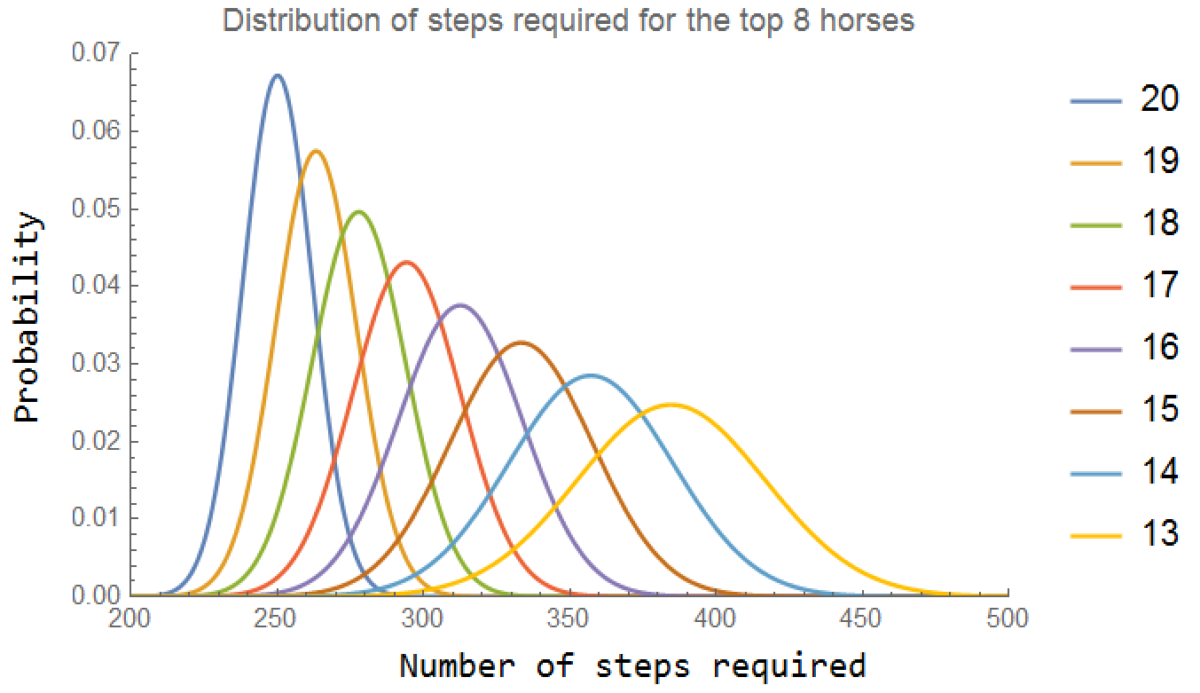# The lucky derbyIn the spirit of the Kentucky Derby, this Riddler puzzle is about a peculiar type of horse race.

The bugle sounds, and 20 horses make their way to the starting gate for the first annual Lucky Derby. These horses, all trained at the mysterious Riddler Stables, are special. Each second, every Riddler-trained horse takes one step. Each step is exactly one meter long. But what these horses exhibit in precision, they lack in sense of direction. Most of the time, their steps are forward (toward the finish line) but the rest of the time they are backward (away from the finish line). As an avid fan of the Lucky Derby, you’ve done exhaustive research on these 20 competitors. You know that Horse One goes forward 52 percent of the time, Horse Two 54 percent of the time, Horse Three 56 percent, and so on, up to the favorite filly, Horse Twenty, who steps forward 90 percent of the time. The horses’ steps are taken independently of one another, and the finish line is 200 meters from the starting gate.

Handicap this race and place your bets! In other words, what are the odds (a percentage is fine) that each horse wins?

Here is my full derivation (long!):
[Show Solution]

For the tl;dr, the answer is:
[Show Solution]

## 6 thoughts on “The lucky derby”

1.Guy Moore says:

Hi Laurent,
This is a very pretty approach. But it doesn’t account for ties, which occur 4.394% of the time.
I looked up the rules of horse racing, and a tie between N horses is treated as 1/N of a win for each horse. Treating ties that way, and performing the discrete Fokker-Planck equations for the probability of each horse being at each step as a function of time, I got slightly different results:
horse 20, 72.394775%
horse 19, 21.69022%
horse 18, 4.97523%
horse 17, 0.832475%
horse 16, 0.0986%
… horse 1, 1.87X10^{-33}%

1.Mike Strong says:

For what it’s worth, my Monte Carlo simulation, which treated ties exactly as you describe, Guy, gave almost exactly the same probabilities as your analytic approach. Very nice!

1.Alden Stowe says:

Hi Mike,

In your Monte Carlo approach how did you accurately simulate the rare event(s) that one of the horses (using Guy’s notation) 1-15 win?

I had a naive simulation and realized quickly that I wasn’t going to be able to accurately distinguish between the probabilities for the uncommon horses winning and 0.

Thanks!

–Alden Stowe

2.Ian Rhile says:

The other way to come up with the formula is to recognize that the final two steps must be forward. Hence, the other 2k-2 steps must be forward and backward steps that net to 2n-2 forward steps. This implies the number of forward “successes” is k+n-2 and backward failures is k-n. Hence,

P(k,n,q) = combin(2*k-2,n+k-2)*q^(n+k-2)*(1-q)^(k-n)*q^2

The last q^2 are the final two steps. I think this is the equivalent of your expression.

3.John Snyder says:

I solved the problem using lattice Green functions as discussed in the book by Hughes, “Random Walks and Random Environments, Vol I”. The following generating function, when expanded as a Taylor’s series about zero, gives coefficients of $z^n$ which are the probabilities that a random walker first reaches the 200 meter finish line after $n$ steps:
$\left(\frac{1-\sqrt{1-4p(1-p)z^2}}{2(1-p)z}\right)^{200}\qquad\text{where }p=0.52,0.54,\dots,0.90.$Using this methodology I incorporated ties up to the fourth order and exactly replicated the probabilities given by Guy Moore.

1.Laurent says:

Neat! thanks! I took the liberty of reformatting your comment using $\LaTeX$. Let me know if there are any typos.